# Interpreting and comparing data distributions

##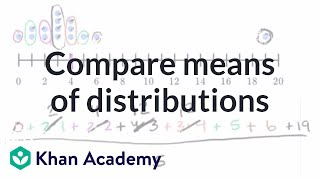By Khan Academy

Interpreting and comparing data distributions# Interpreting and comparing data distributions

##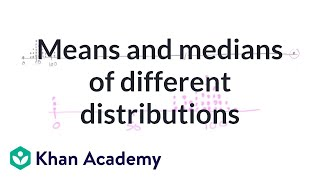By Khan Academy

Interpreting and comparing data distributions# Interpreting and comparing data distributions

##By Khan Academy

Interpreting and comparing data distributions# Interpreting and comparing data distributions

##By Khan Academy

Interpreting and comparing data distributions# Interpreting and comparing data distributions

##By Khan Academy

Interpreting and comparing data distributions# Interpreting and comparing data distributions

##By Khan Academy

Interpreting and comparing data distributions# Reading bar charts: comparing two sets of data

##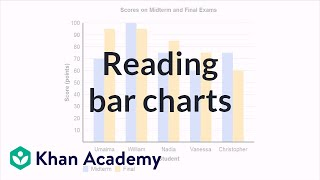By Khan Academy

A bar chart is especially useful with comparing two sets of data. The difference in the bars give us a quick snapshot that allows us to draw some conclusions.# Range, variance and standard deviation as measures of dispersion

##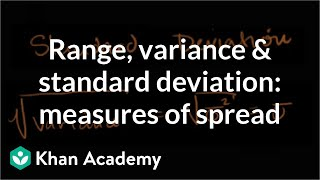By Khan Academy

This video goes over the main ways we measure dispersion of data, then shows those vlaues for two different data sets. Remember that simply comparing the means or medians of two data sets is not sufficient to fully compare the data. Understanding how data is arranged about the "center" of the data is also critical in understand and comparing the sets# Range, variance and standard deviation as measures of dispersion

##By Khan Academy

This video goes over the main ways we measure dispersion of data, then shows those vlaues for two different data sets. Remember that simply comparing the means or medians of two data sets is not sufficient to fully compare the data. Understanding how data is arranged about the "center" of the data is also critical in understand and comparing the sets# Comparing data displays

##By Khan Academy

Sal solves practice problems where he thinks about which data displays would be helpful in which situations.# Ways to Represent Data

##By EducationCommonsRW

This is the second lesson in the series, "Data Handling." The video demonstrates how to compare data using box and whisker plots and how to decide the most appropriate way to represent data graphically.# Basic Rate Problem

##By Khan Academy

This is a basic rate problem where the student is given a table with data and asked to find the rate of travel for 4 trains in order to compare their speed.# Bar Graphs- Pre Algebra Help

##By yourteachermathhelp

Students learn that a bar graph is a visual way to display and compare numerical data (such as the population of different southern states). The bars of a bar graph are drawn in relation to a horizontal axis and a vertical axis and a bar graph can have either vertical or horizontal bars. Students are then asked to create bar graphs using given data and answer questions based on given bar graphs.# Ex 2: Set Problem Solving - Find the Number of Element in the Union of Two Sets Using a Formula

##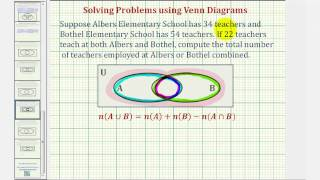By Mathispower4u

Ex 2: Set Problem Solving - Find the Number of Element in the Union of Two Sets Using a Formula# [4.NF.2-1.0] Compare Dissimilar Fractions - Common Core Standard

##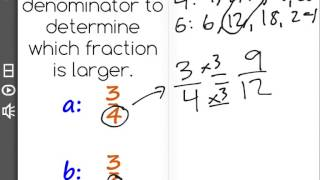By Freckle by Renaissance

Compare two fractions with different numerators and different denominators, e.g., by creating common denominators or numerators, or by comparing to a benchmark fraction such as 1/2. Recognize that comparisons are valid only when the two fractions refer to the same whole. Record the results of comparisons with symbols greater than , = , or less than , and justify the conclusions, e.g., by using a visual fraction model. Front Row is a free, adaptive, Common Core aligned math program for teachers and students in kindergarten through eighth grade. Front Row allows students to practice math at their own pace - learning advanced concepts when they're ready and receiving remediation when they struggle. Front Row provides teachers with access to a detailed data dashboard and weekly email reports that show which standards are causing students difficulty, what small groups can be formed for interventions, and how their students are progressing in math.# [6.RP.1-1.1] Introduction to Ratios - Common Core Standard

##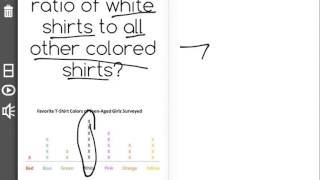By Front Row

Discover more Common Core Math at https://www.frontrowed.comUnderstand that ratios can compare a part to a part or part to a whole or vice versaFront Row is a free, adaptive, Common Core aligned math program for teachers and students in kindergarten through eighth grade. Front Row allows students to practice math at their own pace - learning advanced concepts when they 're ready and receiving remediation when they struggle. Front Row provides teachers with access to a detailed data dashboard and weekly email reports that show which standards are causing students difficulty, what small groups can be formed for interventions, and how their students are progressing in math.Discover more Common Core Math at https://www.frontrowed.com# Number Sets

##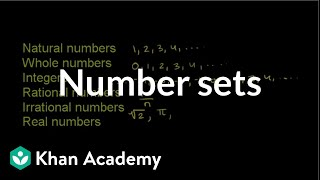By Khan Academy

Number Sets# Constraint solution sets of two-variable linear inequalities

##By Khan Academy

Sal determines which x-values make the ordered pair (x,-7) a solution of 2x-7y<25. He also solves a similar problem where the inequality is given as a graph.# Linear Functions in Practice

##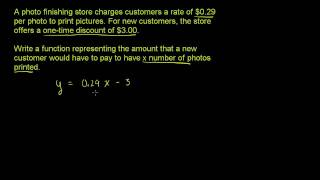By Khan Academy

This video from Khan Academy explores mathematical concepts related to basic linear functions and includes illustrative examples.# Constraint solution sets of systems of linear inequalities

##By Khan Academy

Given the graph of a system of inequalities, Sal finds the x-values that make the ordered pair (x,-2) a solution of the system, which is the solution set constrained to y=-2. Then he solves a similar problem where x is constrained to 4.